Blog

# 5 Ways to Make Collisions (Elastic and Inelastic Forces) A More Approachable Topic

Usama Attiq
Teaching with Labster
October 19, 2022

## Introduction: collision

A collision occurs when two solid objects move toward each other and exert forces on each other for a very short time. The law of moment remains conserved in collisions. It is the interaction between two objects. As a result, the momentum and energy of the colliding objects changes. The collisions are classified into two forces; elastic and inelastic.

The law of moment conservation states that when collisions occur between two objects in an isolated system. The total initial momentum of the objects before the collision is equivalent to the total momentum of the objects after the collision. This law shows that the total momentum of the objects remains conserved. It cannot be changeable or destroyed just like the law of conservation of energy. An isolated system is a system that does not interact with the surrounding environment. In an isolated system, energy and matter cannot exchange with the surrounding environment.

Momentum is defined as the product of the mass of an object and its velocity. It is a vector quantity that has both magnitudes as well as directions. Basically, an object's momentum measures the time required for a force to bring the object to rest.

You should know the difference between interaction and collision. The two objects interact when they are next to each other. While collision is the result of objects coming closer to each other with some velocity and then experiencing an interaction.

In physics, collision is an important topic to understand the basic principle of the law of momentum conservation and kinetic energy. At Labster, we complied with all the complexities that students experience while learning this topic. Then, we provide different ways to make collisions a simpler and easier topic for students to understand. At the end of the topic, we will convince you why a virtual lab simulation is an effective method for teachers to share the topic with their students.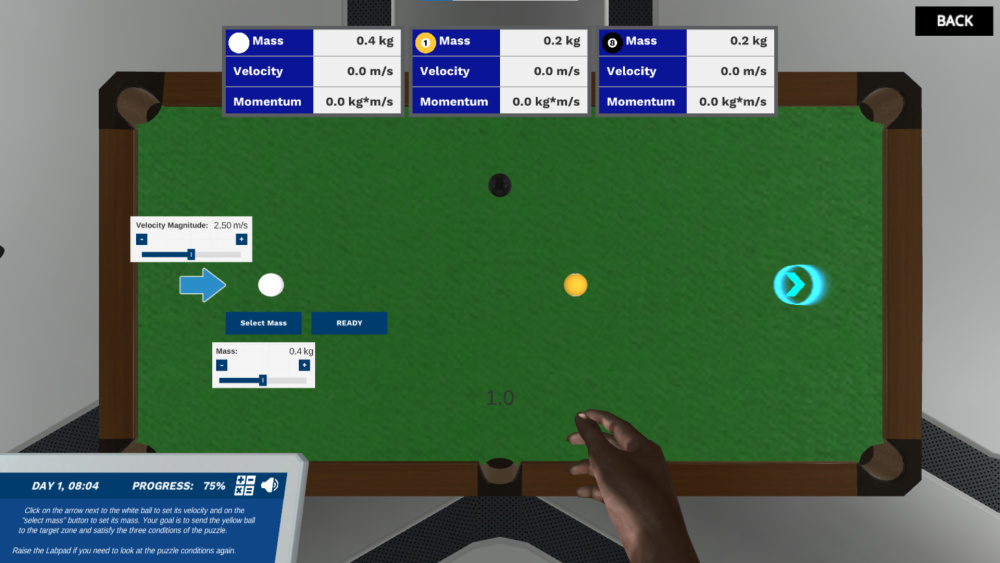Figure: An image explains the collisions from Labster virtual laboratory on Collisions (elastic and inelastic forces)

## Why collisions can be tricky to learn

There are three reasons that make collisions a difficult topic for students to learn.

### 1. It feels abstract

It is hard for students to learn abstract concepts. The main reason is that they cannot see those things with their naked eyes. For instance, if we talk about collisions, there are several abstract terms such as elastic force, inelastic force, momentum, and energy that are used to explain the topic. These are abstract terms because you cannot see them. So, students may find it hard to remember abstract concepts.

### 2. Several mathematical problems

When you learn about collisions, you need to learn many formulas. There are different formulas for both elastic and inelastic collisions. Many students do not have a mathematical representation of elastic and inelastic collisions. They find this topic boring and time-consuming because they need to solve numerical problems. This topic is mainly based on equations that sound difficult for students to understand.

### 3. It’s complicated

In collisions, you must learn several terms and the basic difference between elastic and inelastic collisions. Then, several examples and derivations need to be remembered. In this topic, you have to relate the topic to different other laws present in physics. For instance, you learn about the law of conservation of momentum and kinetic energy and relate them to Newton's second and third laws. Students may get confused while learning about collisions due to the topic's complexities.

## 5 ways to make collisions a more approachable topic to understand

Since we are familiar with the reasons that make collisions hard to understand, five ways make collisions an easier topic to learn.

### 1. Talk about the people behind that science

John Wallis

John Wallis plays an important role in the field of physics. He discovered the concept related to the conservation of momentum. Additionally, he introduced the term “infinity.”  In 1668, a society issued the challenge to produce the theory of the behavior of colliding bodies. Wallis has also participated in this challenge. Wallis and Wren developed the correct theory of conservation of momentum in collision bodies. Later, Wallis did more work to introduce the theory of inelastic collision. He published the three parts of “Mechanica sive De Motu” that described the momentum conservation in elastic and inelastic collisions.

### 2. Basic knowledge of elastic and inelastic forces

Before explaining the types of collisions, the students should know the kinetic energy. Kinetic energy is the type of energy that is produced in an object due to the motion of the object. Collision is classified into two types.

1. Elastic collision

2. Inelastic collision

Elastic collision: Elastic collision is a type of collision in which no loss of kinetic energy (K.E) occurs due to the collision. During an elastic collision, the total kinetic energy and law of conservation of momentum remain conserved and unchanged. This is the reason that the objects can collide without the loss of kinetic energy. Therefore, the total mechanical energy is not transformed into other kinds of energy.

A perfectly elastic collision is a term used in this type of collision. It shows that when two objects have collided, there is no loss of overall kinetic energy. In the case of perfectly elastic collision, the initial and after kinetic energy remains the same and cannot change into other types of energy.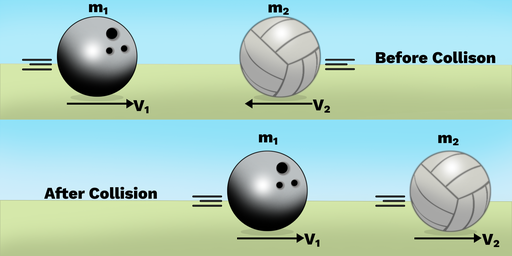Figure: An image shows the elastic collision between a bowling ball and volleyball from Labster theory.

Inelastic collision: In an inelastic collision, the total amount of kinetic energy is changed after the collision from the initial kinetic energy. In this type of collision, the total momentum of the system is conserved but the total kinetic energy is changed. The kinetic energy is converted into other types of energy such as the energy of sound, heat, or internal potential energy.

A perfectly inelastic collision is that in which all the kinetic energy of the objects is converted into other types of energy.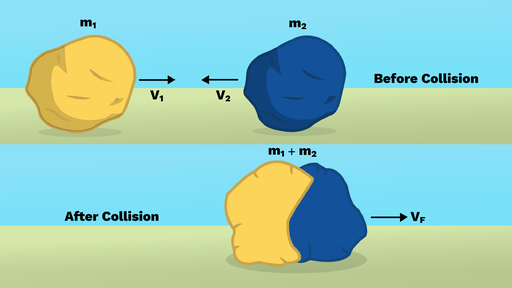Figure: An image of perfectly inelastic collision between mass (m1) and mass (m2) from Labster theory

### 3. Relate to the real world

Students may find learning about elastic and inelastic collisions more interesting when they know this topic's real-world applications. There are very few examples of perfectly elastic and perfectly inelastic collisions in the world.

The balls of the billiards are an example of elastic collisions. When the ball of the billiard strikes another ball, it preserves the momentum and kinetic energy of the system.

Another example of elastic collision is when you throw a ball on the floor, it comes back. The reason is that all the kinetic energy and momentum are conserved when a ball is in motion.

An example of elastic collision is a ballistic pendulum. The ballistic pendulum is a device on which a solid mass is attached to one end. When moving the ballistic pendulum, the kinetic energy is transformed into sound and heat energy. In this example, the pendulum's momentum is preserved, but the kinetic energy is changed into another kind of energy.

Another example of an inelastic collision is a crashed car. When a car is crashed, the kinetic energy is transformed into heat, sound energy, and the distortion of the car.

### 4. Seeing is believing

When they see the color diagrams, students will enjoy learning elastic and inelastic collisions. It helps to transform textbook information into a visual form that is easy to remember. Students are more attracted to color diagrams rather than long text paragraphs. The diverse colors make it simpler to differentiate between the kinetic energy (K.E) before and after collisions. Additionally, learners can remember visual diagrams in the memory appropriately compared to verbal memory.

The images presented below explain the collisions because balls act as objects. When they strike with each other, the initial kinetic energy and, after collision, kinetic energy remain conserved. It means that the total kinetic energy and total momentum remain unchanged and conserved. It shows the example of elastic collision.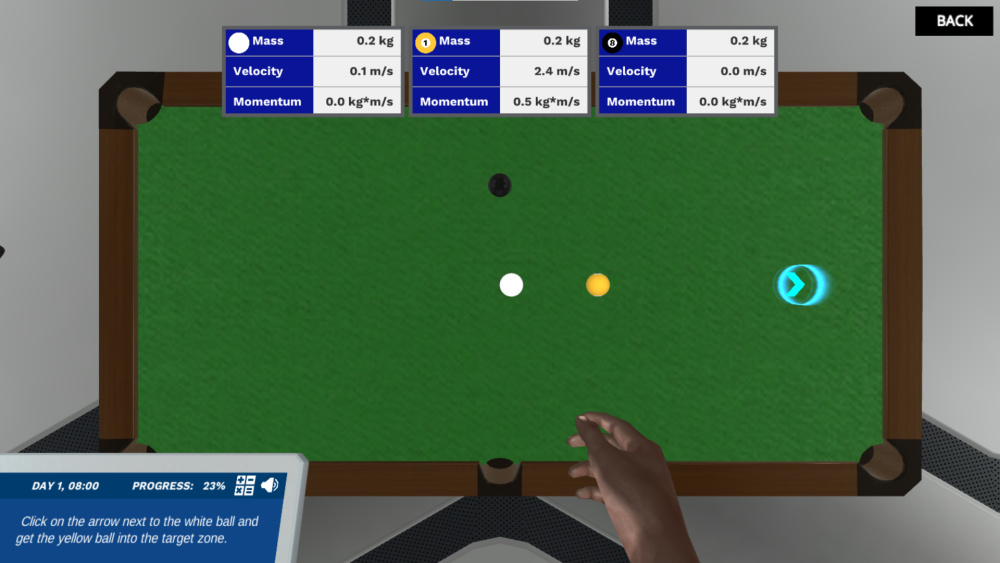Figure: An image explaining elastic collisions from the Labster virtual laboratory on Collisions (Elastic and inelastic forces).

### 5. Use of virtual lab simulation

The virtual lab simulation is an advanced way to teach the students about tough topics like collisions. At Labster, we provide 3D simulations that have gamification elements such as storytelling and a scoring system. These features can help students to understand the topics in the class better.

Labster virtual lab simulation on collisions (elastic and inelastic forces) explains the use of momentum equation to calculate the total momentum before and after the collisions, the law of conservation of momentum, and elastic and inelastic collisions with examples. After using this simulation, the students can understand collisions appropriately.

Check out Labster Collisions Simulation here, or get in touch to find out how you can start using a virtual lab with your students.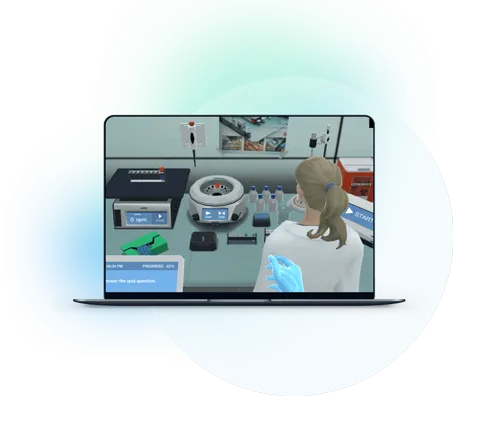What exactly are virtual labs?